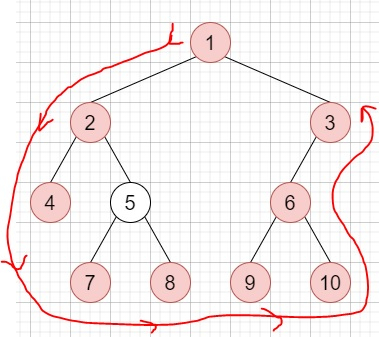# Boundary of Binary Tree in C++

Suppose we have a binary tree, we have to find the values of its boundary in anti-clockwise direction starting from root. Here boundary includes left boundary, leaves, and the right boundary in order without duplicate nodes.

• The left boundary is the path from root to the left-most node.

• The Right boundary is the path from root to the right-most node.

• When the root doesn't have left subtree or right subtree, then the root itself is left boundary or right boundary.

So, if the input is likethen the output will be [1,2,4,7,8,9,10,6,3]

To solve this, we will follow these steps −

• Define an array ret

• Define a function leftBoundary(), this will take node,

• if node is null or node is leaf, then −

• return

• insert value of node into ret

• if left of node is present, then −

• leftBoundary(left of node)

• Otherwise

• leftBoundary(right of node)

• Define a function rightBoundary(), this will take node,

• if node is null or node is leaf, then −

• return

• insert value of node into ret

• if right of node is present, then −

• rightBoundary(left of node)

• Otherwise

• rightBoundary(right of node)

• Define a function leaves(), this will take node,

• if node is not present, then −

• return

• if node is leaf, then −

• insert val of node into ret

• leaves(left of node)

• leaves(right of node)

• From the main method do the following −

• Clear the ret array

• if root is not present, then −

• return ret

• insert val of root into ret

• leftBoundary(left of root)

• leaves(left of root);

• leaves(right of root);

• rightBoundary(right of root)

• return ret

## Example

Let us see the following implementation to get a better understanding −

Live Demo

#include <bits/stdc++.h>
using namespace std;
void print_vector(vector<auto> v){
cout << "[";
for(int i = 0; i<v.size(); i++){
cout << v[i] << ", ";
}
cout << "]"<<endl;
}
class TreeNode{
public:
int val;
TreeNode *left, *right;
TreeNode(int data){
val = data;
left = NULL;
right = NULL;
}
};
void insert(TreeNode **root, int val){
queue<TreeNode*> q;
q.push(*root);
while(q.size()){
TreeNode *temp = q.front();
q.pop();
if(!temp->left){
if(val != NULL)
temp->left = new TreeNode(val);
else
temp->left = new TreeNode(0);
return;
}else{
q.push(temp->left);
}
if(!temp->right){
if(val != NULL)
temp->right = new TreeNode(val);
else
temp->right = new TreeNode(0);
return;
}else{
q.push(temp->right);
}
}
}
TreeNode *make_tree(vector<int> v){
TreeNode *root = new TreeNode(v);
for(int i = 1; i<v.size(); i++){
insert(&root, v[i]);
}
return root;
}
class Solution {
public:
vector<int> ret;
void leftBoundary(TreeNode* node){
if (!node || node->val == 0 || (!node->left && !node->right))
return;
ret.push_back(node->val);
if (node->left && node->left->val != 0)
leftBoundary(node->left);
else
leftBoundary(node->right);
}
void rightBoundary(TreeNode* node){
if (!node || node->val == 0 || (!node->left && !node->right))
return;
if (node->right && node->right->val != 0) {
rightBoundary(node->right);
}
else {
rightBoundary(node->left);
}
ret.push_back(node->val);
}
void leaves(TreeNode* node){
if (!node || node->val == 0)
return;
if (!node->left && !node->right) {
ret.push_back(node->val);
}
leaves(node->left);
leaves(node->right);
}
vector<int> boundaryOfBinaryTree(TreeNode* root){
ret.clear();
if (!root)
return ret;
ret.push_back(root->val);
leftBoundary(root->left);
leaves(root->left);
leaves(root->right);
rightBoundary(root->right);
return ret;
}
};
main(){
Solution ob;
vector<int> v = {1,2,3,4,5,6,NULL,NULL,NULL,7,8,9,10};
TreeNode *root = make_tree(v);
print_vector(ob.boundaryOfBinaryTree(root));
}

## Input

{1,2,3,4,5,6,NULL,NULL,NULL,7,8,9,10}

## Output

[1, 2, 4, 7, 8, 9, 10, 6, 3, ]